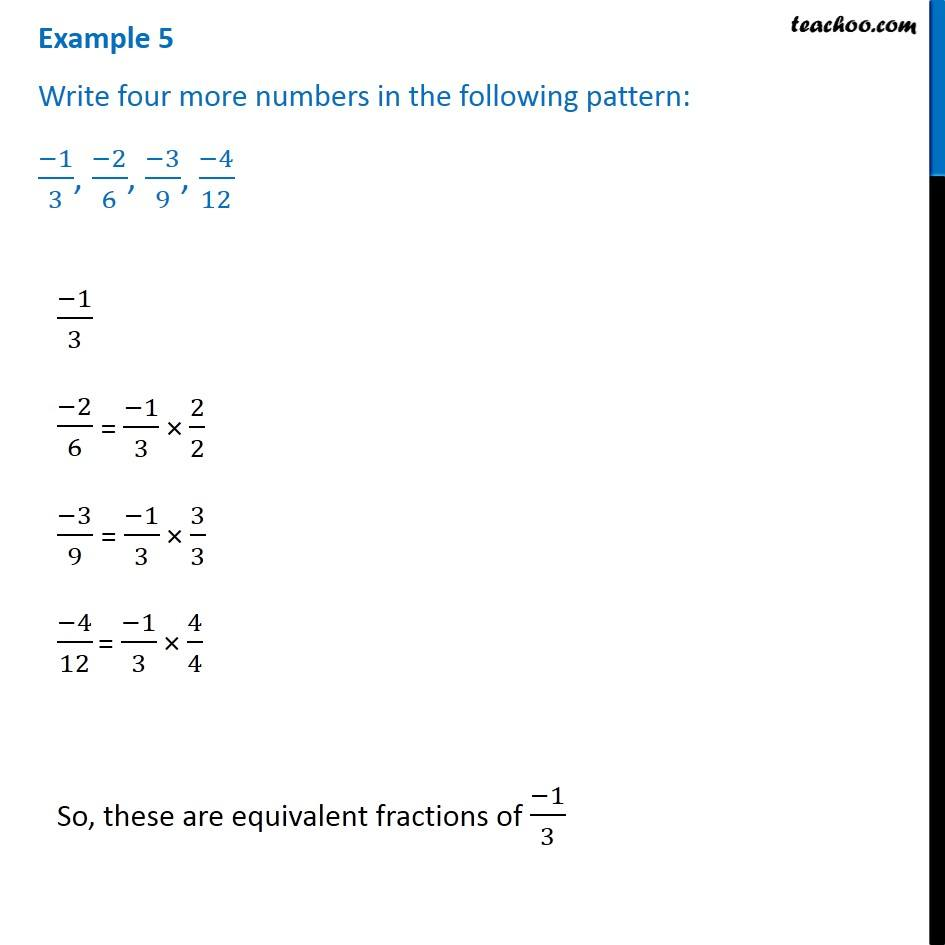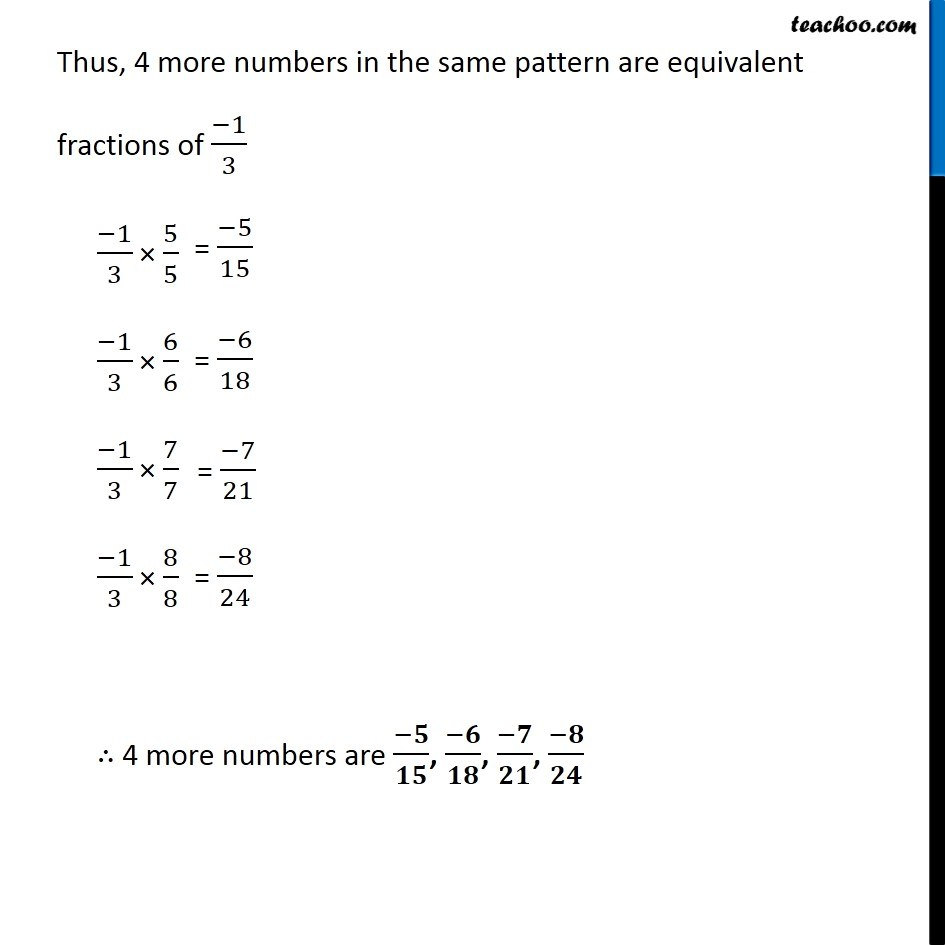Subscribe to our Youtube Channel - https://you.tube/teachoo

1. Chapter 9 Class 7 Rational Numbers
2. Concept wise
3. Equivalent Rational Numbers

Transcript

Example 5 Write four more numbers in the following pattern: (−1)/3, (−2)/6, (−3)/9, (−4)/12 (−1)/3 (−2)/6 (−3)/9 (−4)/12 So, these are equivalent fractions of (−1)/3 Thus, 4 more numbers in the same pattern are equivalent fractions of (−1)/3 (−1)/3 × 5/5 (−1)/3 × 6/6 (−1)/3 × 7/7 (−1)/3 × 8/8 ∴ 4 more numbers are (−𝟓)/𝟏𝟓, (−𝟔)/𝟏𝟖, (−𝟕)/𝟐𝟏, (−𝟖)/𝟐𝟒

Equivalent Rational Numbers

About the AuthorDavneet Singh
Davneet Singh is a graduate from Indian Institute of Technology, Kanpur. He has been teaching from the past 9 years. He provides courses for Maths and Science at Teachoo.